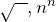# Category: Arithmetic

## Ratios — calculations from the dawn of mathematics

Ratios and fractions are very similar things, since they are both arise from comparing the relative sizes of quantities. Let’s quickly review what exactly a fraction is. If you think of a “whole” as made up of some number of …

## Recurring decimals are cool!

I was thinking about maths teaching the other day (I’m sure you’ll not be surprised to know this is something I often do) and in particular about the different approaches teachers bring to the subject. Teaching maths is a challenge …

## Puzzles with 4 numbers, and the amazing power of powers

A fun puzzle is to choose a number between 1 and 10, then using that number exactly four times in an expression involving basic mathematical operations, i.e. at leastbut also possibly, see what values you can create.

For …